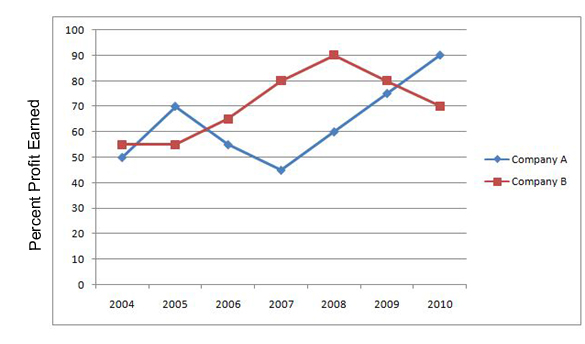## SBI PO DA Test 1

Instructions

Study the following graph carefully to answer the questions.
Percent Profit Earned by Two Companies Producing Electronic Goods over the Years
percent profit =$$\frac{Profit Earned}{Total Investment}\times100$$
Profit Earned = Total Income - Total Investment in the yearQuestion 1

If each of the Companies A and B invested Rs. 25 lakhs in 2010, what was the average profit earned by the two Companies?

Question 2

If the amount invested by the Company B in 2004 is Rs. 12 lakhs and the income of 2004 is equal to the investment in 2005, what was the amount of profit earned in 2005 by Company B?

Question 3

If the investments of the Company A in 2007 and 2008 were equal, what is the difference between profit earned in two years if the income in 2008 was Rs.24 lakhs?

Instructions

For the following questions answer them individually

Question 4

Out of 5 girls and 3 boys, a total of 4 children are to be randomly selected for a quiz contest. What is the probability that all the four children are girls?

Question 5

Amit and Sujit together can complete an assignment of data entry in 5 days. Sujit’s speed is 80% of Amit’s speed and the total key depressions in the assignment are 5,76,000. What Amit’s speed in key depression per hour if they work for 8 hours a day?